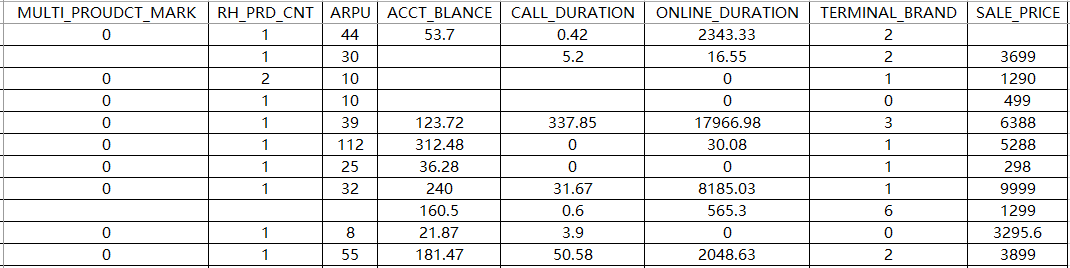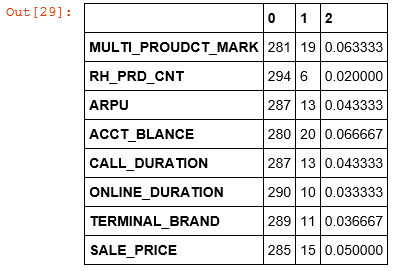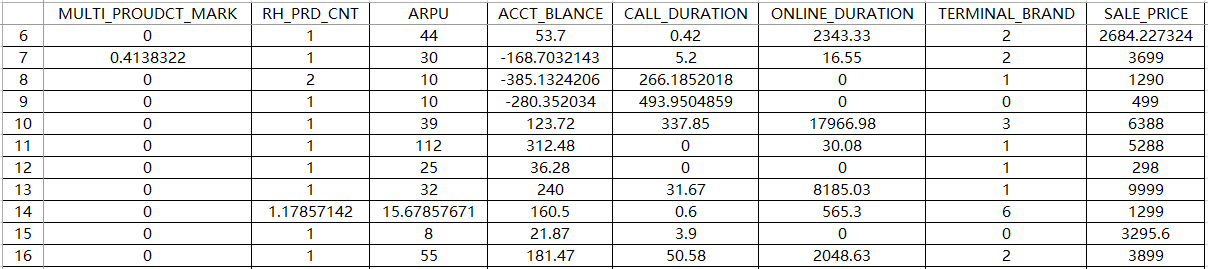• 主要介绍了Python实现的拉格朗日插值法,简单介绍了拉格朗日插值法的原理并结合完整实例形式给出了拉格朗日插值法的具体实现与使用技巧,需要的朋友可以参考下
• python中如何安装SciPY模块Python里面的...~~谁有拉格朗日插值法的python代码啊。急用啊。啦。说不离开的是你，后来一声不吭调头走的也是你。网页链接 Python数据预处理 - 拉格朗日插值法补充缺失值 - 代码实现py...

python中如何安装SciPY模块

Python里面的scipy库如何计算线性规划问题呢？人生的路，靠的是自己一步步去走，真正能保护你的，是你自己的选择。而真正能伤害你的，也是一样，自己的选择。

类似于上述的线性规划问题？~~谁有拉格朗日插值法的python代码啊。急用啊。啦。说不离开的是你，后来一声不吭调头走的也是你。

网页链接 Python数据预处理 - 拉格朗日插值法补充缺失值 - 代码实现

python 拉格朗日插值 不能超过多少个值

拉格朗日插值Python代码实现 1. 数学原理 对某个多项式函数有已知的k+1个点，假设任意两个不同的都互不相同，那么应用拉格朗日插值公式所得到的拉格朗日插值多项式为： 其中每个lj(x)为拉格朗日基本多项式(或称插值基函数)。

什么是维纳滤波，对于python里的函数scipy.signal

什么是维纳滤波，对于python里的函数scipy.signal.wiener(im,mysize=non文档很细致埃 im : ndarray An N-dimensional array. mysize : int or arraylike, optional A scalar or an N-length list giving the size of the Wiener filter window in each dimension. Elements of mysize should be odd. If mysize is a

不要再用python IDLE 了，那样你会在现在的环境下陷入绝望的，使用Anaconda吧，只要把anaconda用好，安装什么的不用再找包了。

如何使用jython调用python的scipy和numpy

以上就是四十五资源网为大家整理的python中的插值 scipy-interp的实现代码 python 拉格朗日插值 不能超过多少个值内容,如果觉得本站更新的资源对您有帮助 不要忘记分享给您身边的朋友哦！

展开全文• 拉格朗日插值Python代码，用于处理Excel中数据缺失。简单的算法
• python拉格朗日插值（详细）

万次阅读 2017-12-08 15:41:53
在若干个不同的地方得到相应的观测值,拉格朗日插值法可以找到一个简单函数,其恰好在各个现测的点取到观测到的值。本文基于Python实现一个简单的拉格朗日插值

拉格朗日插值简单介绍

拉格朗日插值法是以法国十八世纪数学家约瑟夫·拉格朗日命名的一种多项式插值方法。

许多实际问题中都用函数来表示某种内在联系或规律，而不少函数都只能通过实验和观测来了解。在若干个不同的地方得到相应的观测值,拉格朗日插值法可以找到一个简单函数,其恰好在各个现测的点取到观测到的值,这个函数可以是代数多项式,三角多项式等。

本文基于Python实现一个简单的拉格朗日插值。

# -*- coding: utf-8 -*-

# 拉格朗日插值实现
# time:2017.11.01

读取数据

import pandas as pd
inputfile = "E:\\Python\\model1\\train_test2.csv"
train_data = pd.read_csv(inputfile, encoding = 'utf-8')

部分数据展现查看数据集大小

print('数据量:',train_data.shape)

数据量: (300, 8)

查看缺失值情况

def na_count(data):
data_count = data.count()
na_count = len(data) - data_count
na_rate = na_count/len(data)
result = pd.concat([data_count,na_count,na_rate],axis = 1)
return result;
na_count(train_data)拉格朗日插值

import pandas as pd
from scipy.interpolate import lagrange
def ployinterp_column(s,n,k=5):
y = s[list(range(n-k,n)) + list(range(n+1,n+1+k))]
y = y[y.notnull()]
return lagrange(y.index,list(y))(n)

逐个元素判断是否需要插值

for i in train_data.columns:
for j in range(len(train_data)):
if (train_data[i].isnull())[j]:
train_data[i][j] = ployinterp_column(train_data[i],j)

输出结果

outputfile = "E:\\Python\\model1\\train_test_out.xls"
train_data.to_excel(outputfile)

查看数据展开全文python 拉格朗日插值 缺失值
• 拉格朗日插值代码(缺失值的数据处理)import pandas as pd #导入数据分析库Pandasfrom scipy.interpolate import lagrange #导入拉格朗日插值函数inputfile = 'F:/python 数据挖掘分析实战/Data/catering_sale.x...

A.数据源：catering_sale.xlscatering_sale.xls

A.拉格朗日插值代码(缺失值的数据处理)

import pandas as pd #导入数据分析库Pandas

from scipy.interpolate import lagrange #导入拉格朗日插值函数

inputfile = 'F:/python 数据挖掘分析实战/Data/catering_sale.xls' #销量数据路径

outputfile = 'F:/python 数据挖掘分析实战/Data/tmpsales.xls' #输出数据路径

data[u'销量'][(data[u'销量'] < 400) | (data[u'销量'] > 5000)] = None #过滤异常值，将其变为空值，即变为缺失值 nan

#自定义列向量插值函数

#s为列向量，n为被插值的位置，k为取前后的数据个数，默认为5

def ployinterp_column(s, n, k=5):

y = s[list(range(n-k, n)) + list(range(n+1, n+1+k))] #取数

y = y[y.notnull()] #剔除空值

return lagrange(y.index, list(y))(n) #插值并返回插值结果

#逐个元素判断是否需要插值

for i in data.columns:

for j in range(len(data)):

if (data[i].isnull())[j]: #如果为空即插值。

data[i][j] = ployinterp_column(data[i], j)

data.to_excel(outputfile) #输出结果，写入文件

B. 数据源：normalization_data.xlsnormalization_data.xls数据规范化结果

数据代码

import pandas as pd

datafile = 'F:/python 数据挖掘分析实战/Data/normalization_data.xls' #参数初始化

(data - data.min())/(data.max() - data.min()) #最小-最大规范化

(data - data.mean())/data.std() #零-均值规范化

data/10**np.ceil(np.log10(data.abs().max())) #小数定标规范化

参考资料：《Python数据分析与挖掘实战》

展开全文• 利用python中matplotlib库，实现绘制牛顿插值、拉格朗日插值、三次样条插值函数图像。 随机在图像上产生5个随机点，用三种插值方式求函数，并用python中matplotlib库绘制在图像上
• 运用拉格朗日插值法给空缺数据进行插值，通过调用scipy中的lagrange实现
• 本文主要涉及两个内容1、拉格朗日插值的数学理论2、Python实现拉格朗日插值1、拉格朗日插值对于所取得的n个样本数据(xi, yi)(其中n=1,2，...,n)，满足的n次多项式，然后计算多项式在兴趣点的值，把这种方法叫做...

一般在数据处理的过程中，会遇到缺失值的情况，对于缺失值的处理一般会删除或者插值补充。其中拉格朗日插值就是一种最简单的插值方法。本文主要涉及两个内容

1、拉格朗日插值的数学理论

2、Python实现拉格朗日插值

1、拉格朗日插值

对于所取得的n个样本数据(xi, yi)(其中n=1,2，...,n)，满足的n次多项式，然后计算多项式在兴趣点的值，把这种方法叫做拉格朗日插值，其实就是解方程组。对于有n个数据样本，需要n-1次多项式函数，将n个数据带入函数中，得到n元一次的方程组，解出方程组就能得到函数。于是就能计算你感兴趣的x对应的y值了。

n-1次多项式函数为

![](http://www.forkosh.com/mathtex.cgi? \large y=\sum_{i=0}{n-1}a_ixi)

将n个样本数据带入以上方程中得到：

![](http://www.forkosh.com/mathtex.cgi? \large \mathbf{Y}=\mathbf{XA})

其中：方程的解的问题就转化为矩阵X是否可逆，如果可逆那么方程就有解。而解的个数取决于X的秩，如果X的秩为n有唯一解，X的秩小于n，方程就有无数个解。

2、Python实现拉格朗日插值

只需要导入scipy.interpolate的lagrange方法

# -*- coding:utf-8 -*-

from scipy.interpolate import lagrange

import numpy as np

import matplotlib.pyplot as plt

def interp_lagrange(x, y, xx):

# 调用拉格朗日插值，得到插值函数p

p = lagrange(x, y)

yy = p(xx)

plt.plot(x, y, "b*")

plt.plot(xx, yy, "ro")

plt.show()

if __name__ == '__main__':

NUMBER = 20

eps = np.random.rand(NUMBER) * 2

# 构造样本数据

x = np.linspace(0, 20, NUMBER)

y = np.linspace(2, 14, NUMBER) + eps

# 兴趣点数据

xx = np.linspace(12, 15, 10)

interp_lagrange(x, y, xx)根据20个样本点插值结果

注意

1、很多人可能觉得样本数据越多，得到的插值数据会越精确，这样想法是不正确的。理论上说，样本数据过多，得到的插值函数的次数就越高，插值的结果的误差可能会更大。拉格朗日插值的稳定性不太好，出现不稳定的现象称为龙格现象，解决的办法就是分段用较低次数的插值多项式。

2、插值一般采用内插法，也就是只计算样本点内部的数据。

展开全文• #拉格朗日插值代码 import pandas as pd #导入数据分析库Pandas from scipy.interpolate import lagrange #导入拉格朗日插值函数 inputfile = '../data/catering_sale.xls' #销量数据路径 outputfile = '../tmp/...
• import pandas as pd from scipy.interpolate import lagrange inputfile = r'missing_data.xls' outputfile = r'new.xlsx' df = pd.read_excel(inputfile, header=None) print(len(df)) print(len(df.columns)) ...
• 关于拉格朗日插值算法的定义可以查看维基百科拉格朗日插值法。 这里直接上代码，当前代码不是最优算法 class Algorithm: @staticmethod def LagrangeInterpolation(): grid_x = np.array([4, 5, 6]) # the ...算法 python
• 拉格朗日插值法代码与实现 import matplotlib.pyplot as plt import numpy as np import math import random y_point = [] text_list = [] x_point = random.sample(range(0,12),10)#生成不重复的随机数 for num in...python
• from scipy.interpolate import lagrange # 导入拉格朗日插值函数 def ployinterp_column(s, n, k=5): y = s.iloc[list(range(n-k, n)) + list(range(n+1, n+1+k))] # 取数 y = y[y.notnull()] # 剔除空值 ...python 数据分析 机器学习
• 拉格朗日插值法，如何计算数值？ 二、详解拉格朗日插值算法 插值法就是利用已知的点(x,y)建立合适的插值函数y=f(x),将未知点w带入插值函数 f(x)可以求出函数值 f(w)。对于平面上相异（无两点在一条直线上）的 n个点...算法 数据分析 大数据 python 人工智能
• 拉格朗日插值实现及求解 输入：插值节点与插值节点的函数值，以及所要求近似值的x值; 输出：拉格朗日插值多项式（符号函数），x处的函数近似值. def Lagrange(xx,yy,a): import sympy as sym from fractions ...python
• 插值法是数值分析的基础知识之一，本文介绍的拉格朗日插值法是一种多项式插值法，可以用于数据不完整时的填补工作，本文包含理论介绍和python实现两个部分。1、什么是插值问题？假设自己拥有下面的数值序列，由于...
• python代码 import numpy as np X = input("x的值：").split(' ') Y = input("y的值：").split(' ') x = input("要预测的值：") X = np.array(X).astype(np.float64) Y = np.array(Y).astype(np.float64) x = np....
• 拉格朗日插值法python实现

千次阅读 2021-01-03 14:11:26
对某个多项式函数有已知的k+1个点，假设任意两个不同的都互不相同，那么应用拉格朗日插值公式所得到的拉格朗日插值多项式为： L(x)=∑j=0kyjℓj(x) L(x)=\sum_{j=0}^{k} y_{j} \ell_{j}(x) L(x)=j=0∑k​yj​ℓj​(x...python
• Python实现的拉格朗日插值法

千次阅读 多人点赞 2020-03-27 00:40:11
#拉格朗日插值代码 import pandas as pd #导入数据分析库Pandas from scipy.interpolate import lagrange #导入拉格朗日插值函数 inputfile = 'catering_sale.xls' #销量数据路径 data = pd.read_excel(inputfile) #...python
• 已知sinx的一组x,y对应关系,用拉格朗日插值法估计sin(0.3367)的值.xx0.320.340.36y0.3145670.3334870.352274//class Interpolation:def __init__(self, x, y):self.x = xself.y = ydef func(self, X):s = 0for i in ...python插补法
• 前言 学习需要。 正文 1. 拉个朗日插值法 1.1 程序 #!/usr/bin/env python # -*- coding: utf-8 -*- import numpy as np import matplotlib.pyplot as plt import time #print(plt.__file__ ) ... """拉格朗日插值...
• 由于做作业懒得动手算，于是花了更多的时间编了个小程序。 程序 import numpy as np class Lagrange(): def __init__(self,X,Y,n): self.X=*n for i in range(n): X_copy=X.copy() del X_copy[i] ...python
• 下面即为拉格朗日插值法的一个实例 我将文件上传到，我的资源当中了，可以直接下载，运行 import pandas as pd from scipy.interpolate import lagrange #导入拉格朗日插值函数## #缺失值处理——拉格朗日插值法 ...python
• 拉格朗日插值代码python实现（不掉包） 今天我们来讲一下，使用拉格朗日插值公式进行插值，通过python实现 那么拉格朗日插值公式是什么样的呢？ 百度百科定义如下： 当然如果你没有看懂的话，可以再去查询一些相关...python 算法...

python拉格朗日插值python 订阅